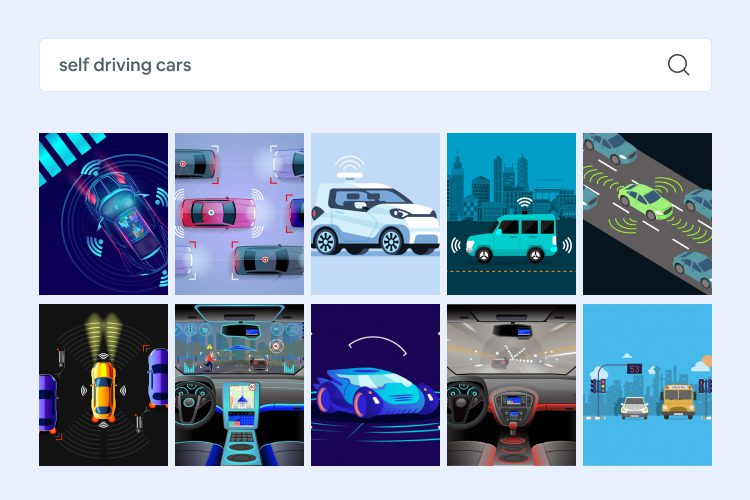#### Text-Based Image Retrieval: Using Deep Learning

June 10, 2021

Text-based image retrieval (TBIR) systems use language in the form of strings or concepts to search relevant images. Computer Vision and Deep Learning algorithms analyze the content in the query image and return results based on the best-matched content. With the rapid advancement in Computer Vision and Natural Language Processing(NLP), understanding the semantics of text and images becomes mandatory as Computer Vision trains computers to interpret and understand the visual world whereas NLP gives machines the ability to read, understand, and derive meaning from human languages. These machines accurately identify and classify objects by using digital images and analyze them with deep learning models.

With Computer Vision and Natural Language Processing(NLP) gaining a lot of momentum in the recent technological developments, cross-modal network learning for image-text similarity plays a very important role in the task of a query(image/text) based image retrieval as the application of image-text semantic mining can be harnessed using the cross-modal network. This blog focuses on text or referral, expression-based queries to rank and retrieve semantically similar images.

We attempted to explore the semantic similarity between the visual and text features that can be used to further research on object and phrase localization areas by building a cross-modal similarity network that uses Triplet loss to train and use Cosine similarity to generate K-similarity scores for a given text query. The dataset that we used for this problem is the Flickr8k dataset.

Let’s dive into building the machine learning pipeline that involves

– Data understanding and preparation
– Image and text embedding extraction
– Similarity network
– Triplet loss
– Training and evaluation

## Data understanding and preparation

We are using Flickr dataset as it is smaller and the image resolution considered is 224X224X3. Flickr dataset has two sections containing the images and their corresponding matching captions. The dataset contains a total of 8092 images in JPEG format with different shapes and sizes, of which 6000 are used for training, 1000 for the test, and 1000 for development. Flickr8k text contains text files describing the training set, test set. Flickr8k.token.txt contains 5 captions for each image i.e. total of 40460 captions.

Below are the code snippets for loading the data, checking the number of images and number of captions per image, plotting the image with its corresponding captions for 4 images.

– The total number of images is 8091.
– The number of captions per image is 5.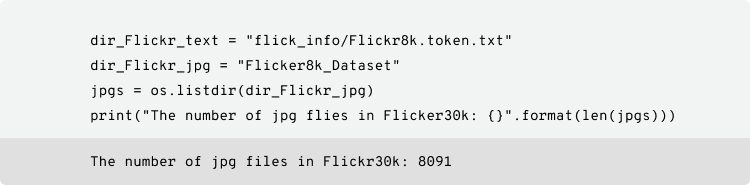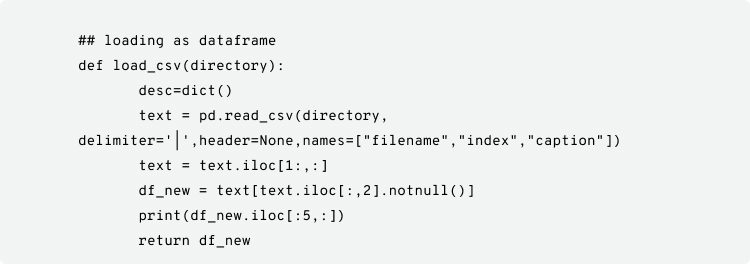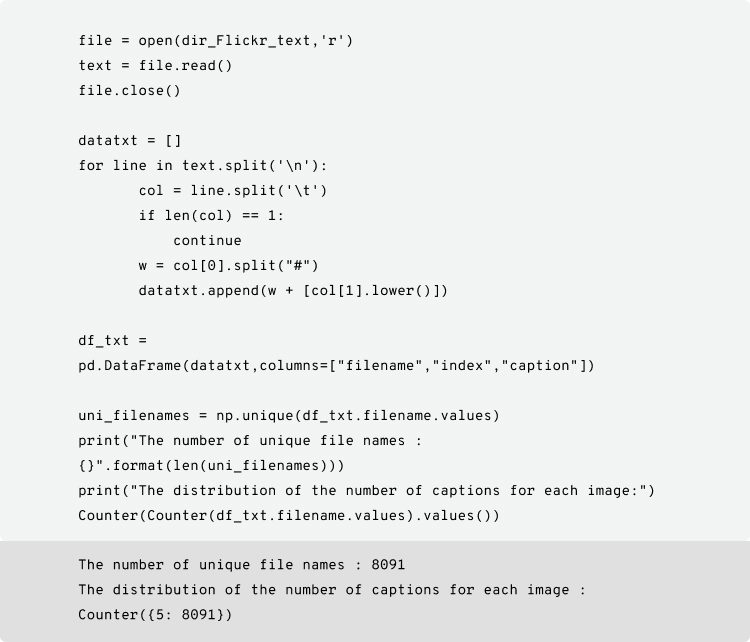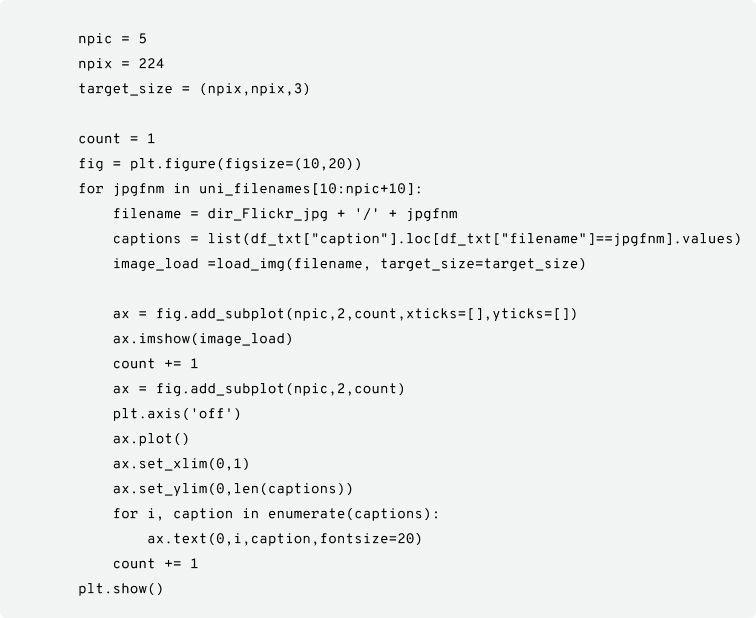The plot of 4 random images with corresponding captions are shown below:

##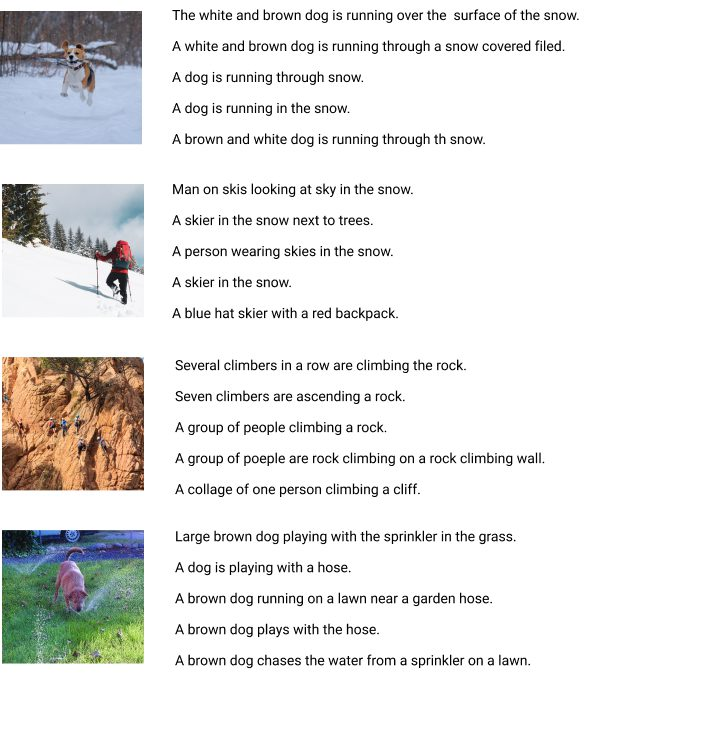Get image embedding

Extraction of visual features is done by using a pre-trained model on ImageNet. ImageNet pretraining is an auxiliary task in Computer Vision problems. It is formally a project aimed at manually labeling and categorizing images into almost 22,000 separate object categories for the purpose of Computer Vision research. The state-of-the-art pre-trained networks included in the Keras core library demonstrate a strong ability to generalize images in real-life scenarios via transfer learning, such as feature extraction and fine-tuning.

There are various pre-trained models like VGG-19, RESNET, etc. Here we are using ResNet 50 for getting the image vectors. We need only the embedding vector, so we will not be using the entire network. Instead, we will use it until the “conv5_block3_3_conv” layer finally gives a 2048 sized embedding.

Below is the code snippet for extracting the image embeddings.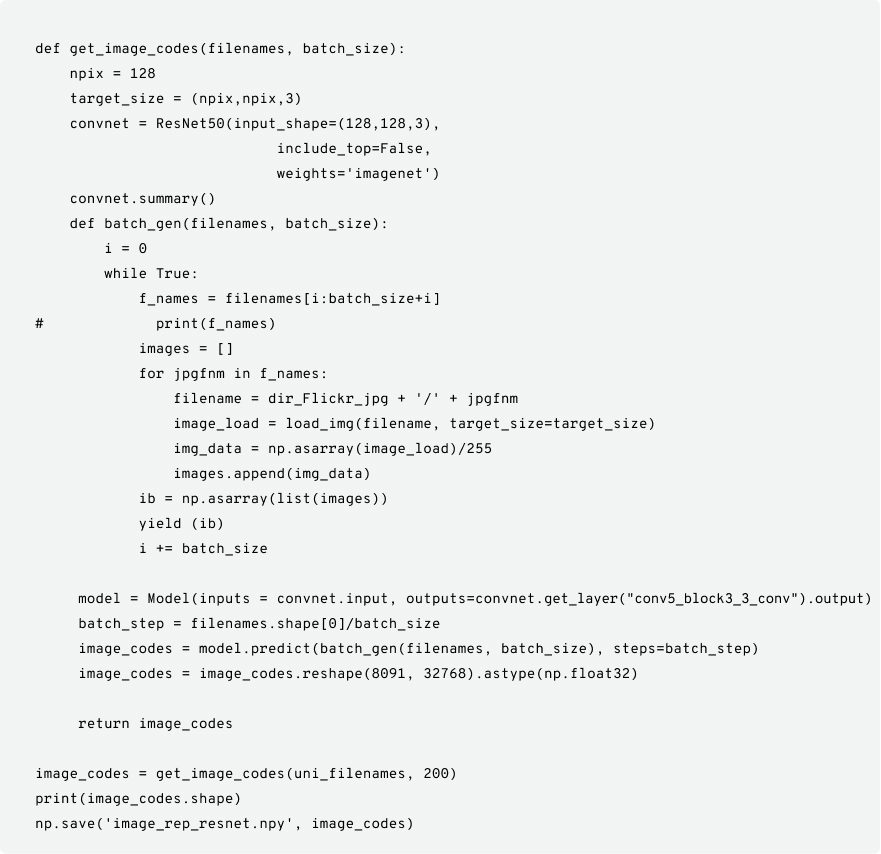In order to further optimize the performance of the model, instead of loading the entire image into the embedding model let us try and create mini-batches that yield a pool of image sets. Online preprocessing is applied on these mini-batches by converting the image into an n-dimensional array of pixel values, which are further normalized and passed into the CNN model that we considered from ResNet50 for prediction. The resultant output is the image embedding for the pool of images generated by the mini-batch creator. Online batch creation is the idea of yielding the preprocessed images on the fly based on the batch size mentioned.

## Get text embedding

For text embedding, individual words are represented as real-valued vectors in a predefined vector space where each word is mapped to one vector. The text embedding technique is often merged into the field of Deep Learning as the vector values are learned in a way that resembles a neural network. The distributed representation, based on the usage of words, captures their meaning by allowing words used in similar ways to result in having similar representations.

There are various pre-trained models, like Glove, SkipThoughts, etc, to get text embedding performed. The model we are considering is the Glove pre-trained model that derives the relationship between the words. It considers the global statistics-GloVe 300-dimensional vectors trained on the 42B token common crawl corpus.

The idea is to create a representation of words that capture the meanings, semantic relationships, and the contexts that they are used in. This will help us to achieve transfer learning. Transfer learning could be either about the words or about the embedding. Our main area of concentration is to obtain the embedding for the provided input captions.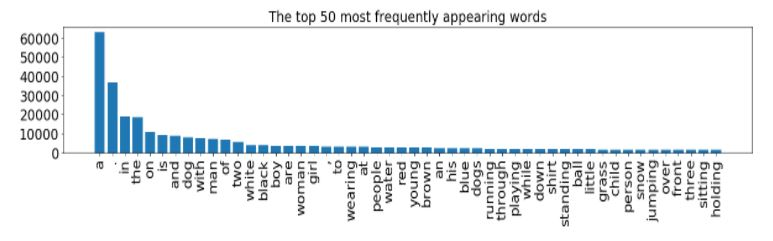There are four functions used in this pipeline to extract the vectors of captions:

– loadGloveModel( ) – This function loads a pre-trained word embedding dictionary of 40000 words with 300 sized vectors each.
– Cap_tokenize(captions) – This function splits the sentences into tokens (words), removes punctuations, single letters, and finally joins them back as sentences.
– Text2seq (tokenized_captions) – This function converts captions into sequences of integers using Tensorflow functions like pad_sequences and text_to_sequences. The max vocabulary size is fixed as 4500 and the max length of any sequence is set as 30.
1. First, create a Tokenizer object with a vocabulary size of 4500.
2. The object is then trained on cleaned captions that return a word dictionary with an index based on the frequency of word occurrence in descending order.
3. Now, the captions are converted to integer sequences.
4. Use pad_sequences to have a max of 30 sequences in one row.
5. Create an embedding matrix of size (Vocab_size, 300 ), where 300 is the word vector dimension.

This function returns the word dictionary, tokenizer object, embedding matrix, and caption converted as sequences.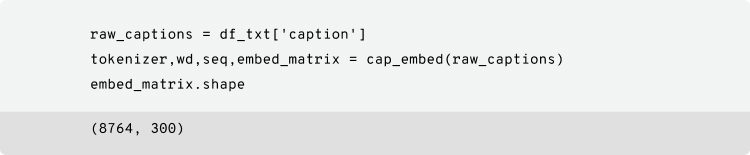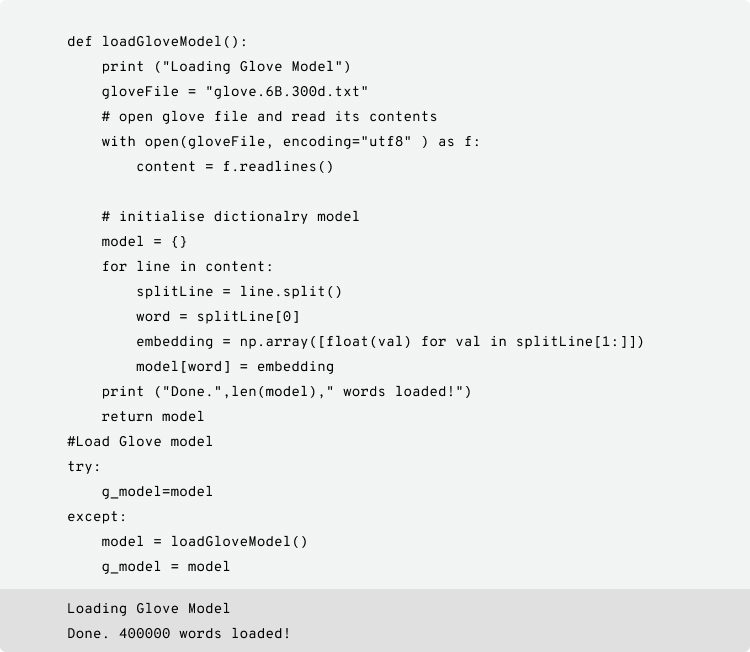Below are the functions for text preprocessing and conversion of text to sequences: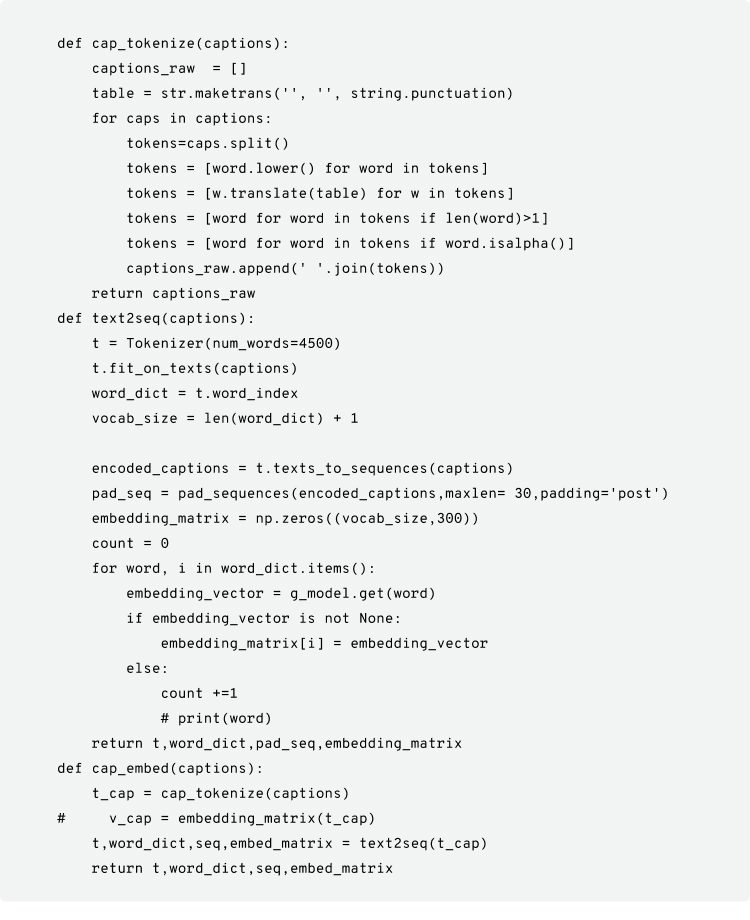## Building Siamese network with triplet loss (similarity network)

A Siamese network, an artificial neural network, computes comparable output vectors by using the same weight while working sequentially in two different input vectors. Learning in such a kind of a twin network can be done by considering the triplet/contrastive loss function.

The similarity network with triplet loss accepts three branches of input – consisting of an anchor image, a negative caption, and a positive caption in the embedded form. This network is an improvised version of a contrastive loss model, where if given a single input, it learns about the correct pair of image and text as well as learns which of the image-text pairs are not suited.

Each branch will be passed through a series of transformations (applied by fully connected layers separated by rectified linear unit non-linearities) which will output the similarity scores based on the Euclidean distance metric, after the conversion to modality robust features. The training objective is to minimize the triplet loss function that applies a margin-based penalty to an incorrect caption when it gets ranked higher than the correct one describing the anchor image similarly for the given positive caption, i.e., the image gets ranked higher than the unrelated images.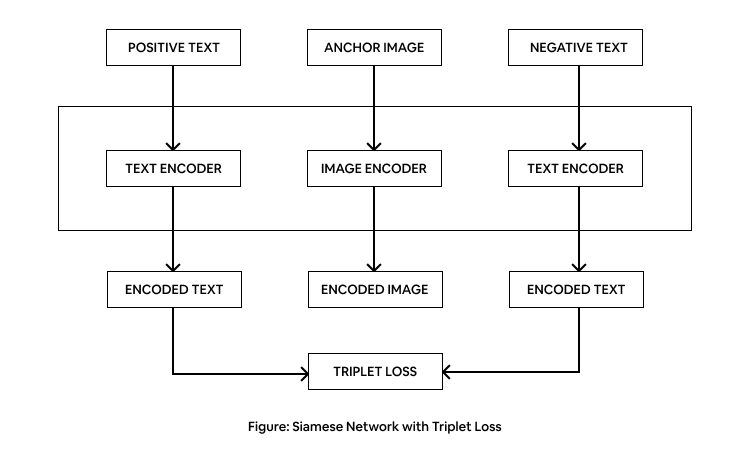Below is the model for the image encoder: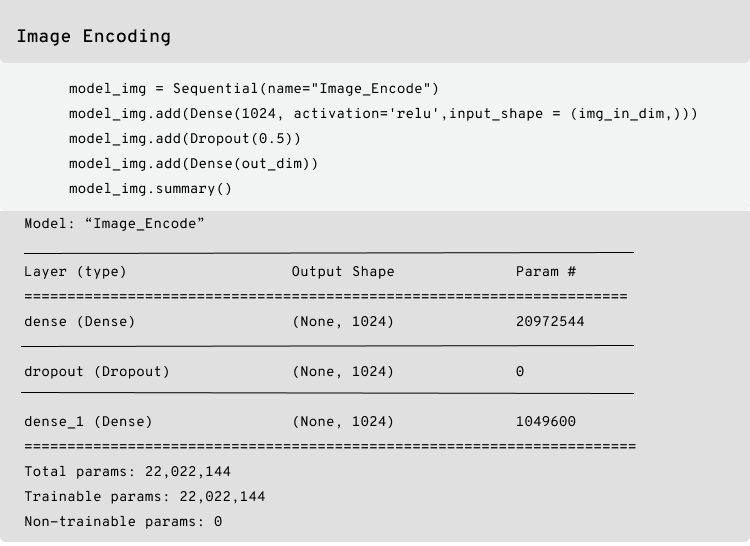Below is the model for text encoder: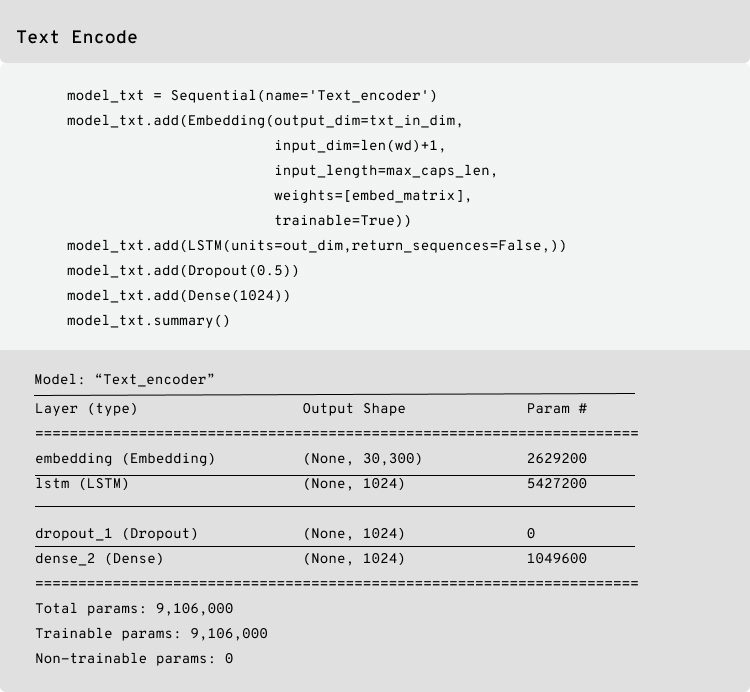Below is the Siamese model: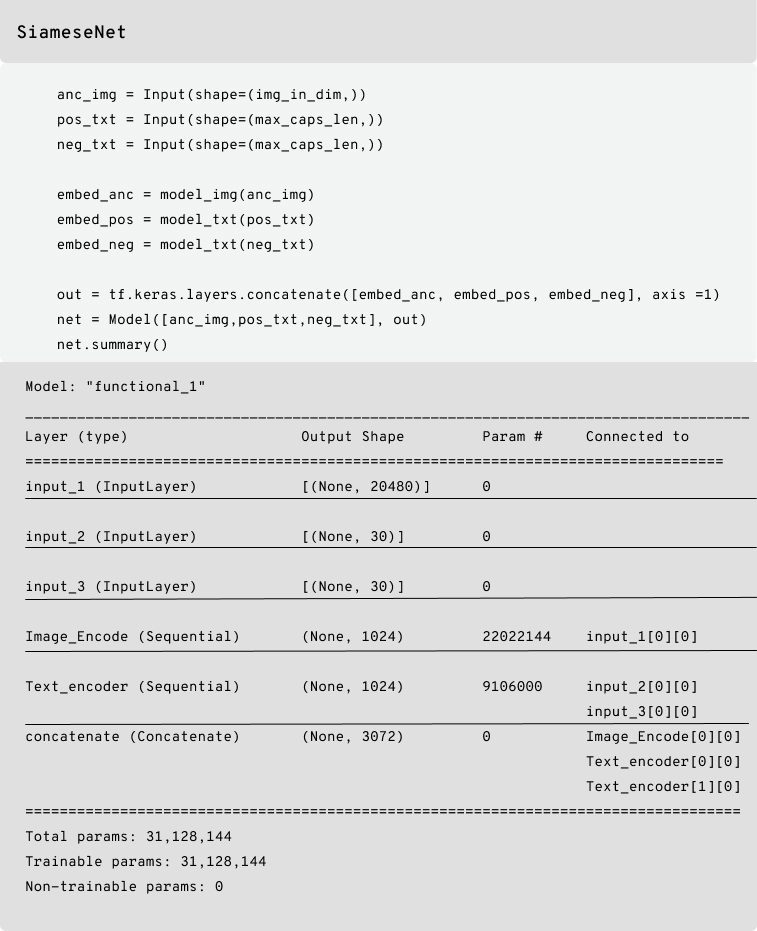## Triplet batching and Triplet loss

Given the anchor image “a”. Let p and n be the matching positive caption and the non-matching negative caption. We will calculate the euclidean distance between D1 (a, p) and D2 (a, n). We want the D1 value to be smaller than the distance value D2 and according to the definition of triplet loss, each negative caption “n” should be enforced with a margin of “m” which is called to be neighborhood updation. Based on the definition of triplet loss there are various options of selecting the triplets namely:
– Easy triplets
– Semi-hard triplets
– Hard triplets

An easy triplet has a loss of zero because D(a, p) + m will be less than D(a, n). Whereas, hard triplets are the ones in which the negative caption is closer to the anchor image than the positive caption because D(a, n) is less than D(a, P). Semi-hard triplets are the ones where the negative caption is not closer to the anchor image than the positive caption – which still has a positive loss associated with it because D(a, p) is less than D (a, n) which is less than D(a, p) + m.

The ultimate goal is – given a caption, a pool of images, and given a K value for recall, the model should be able to retrieve the most relevant images with lower loss.

Below is the code snippet for Triplet batching function (Easy triplets) and Triplet loss function-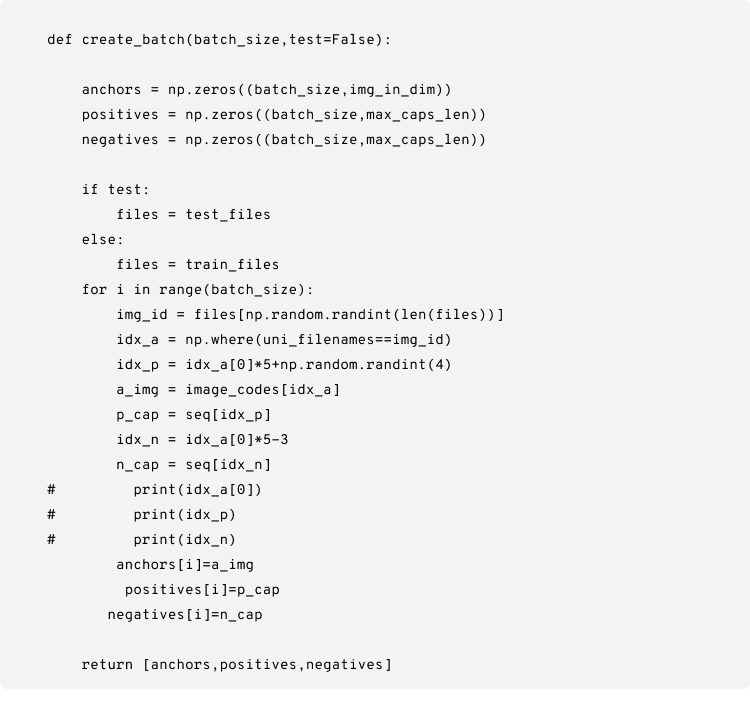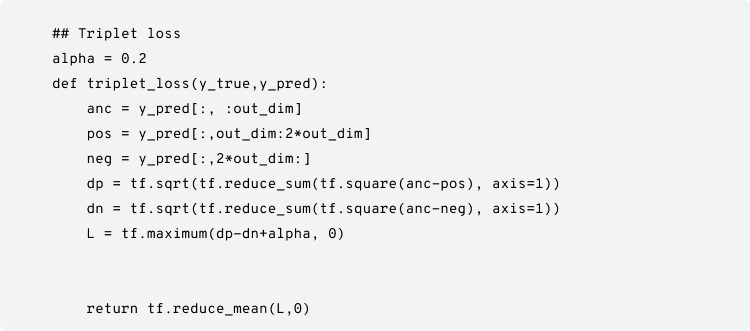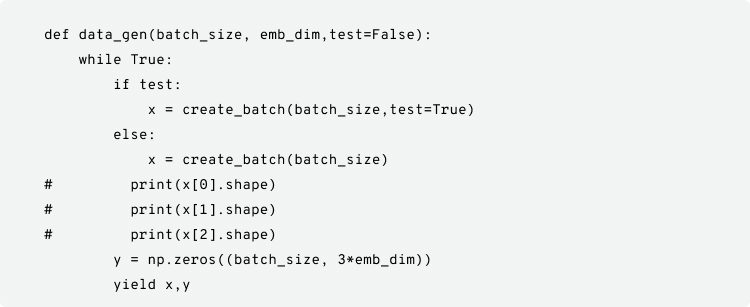## Training the Similarity network

Now that we have built our siamese network and triplet dataset, it is time to train our model. The data consist of 8091 images with 5 captions per image and out of which, 6091 images and their captions are used for training.

The training parameters are: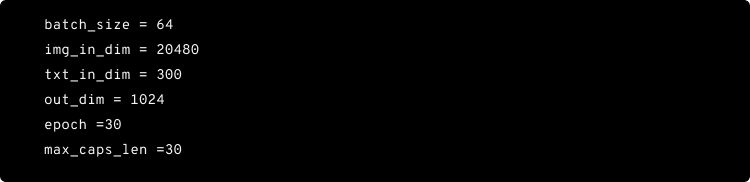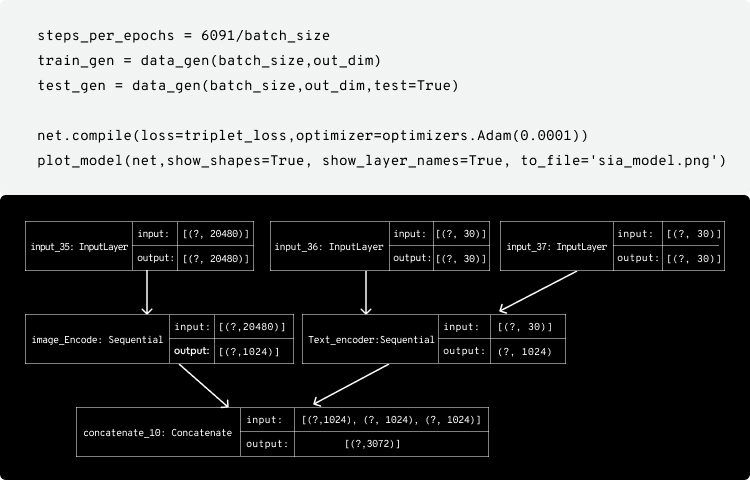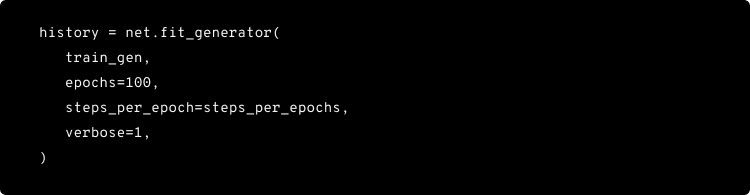Following is the training loss plot :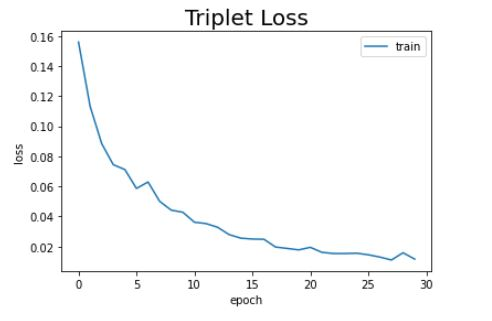## Evaluation

As our model is now trained to understand the semantic similarity between an image and its corresponding captions, it is time to evaluate the model with test cases. For that, it is necessary to build a function user_input_text_embed( ) :

– The user can give any text query. The text is converted to a tokenized integer sequence. For this we use the same Tokenizer object we trained on train captions.
– The tokenized sequence is given to text_encode_model to predict its text embedding.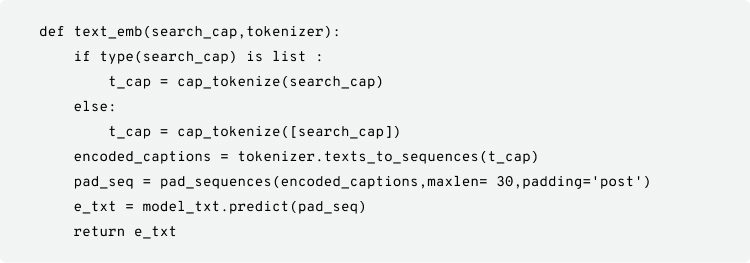These are some text inputs –

## Cosine Similarity Function:

We are now using cosine similarity to find the K-nearest image embeddings to the given text embedding.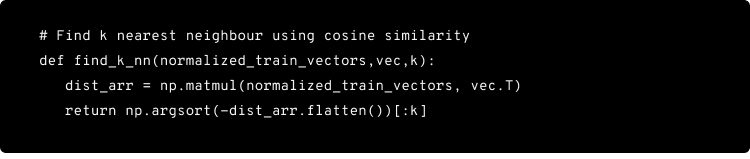Let us keep K =10, which means to output the top 10 images for a given text query.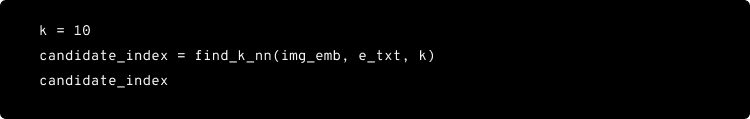Candidate index contains the index of 10 images :
Example: array([7778, 5830, 6500, 6534, 2893, 6290, 6354, 3239, 6948, 7762])

### Find the corresponding images using the indices: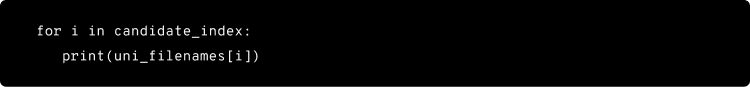Plotting Top K images in a grid: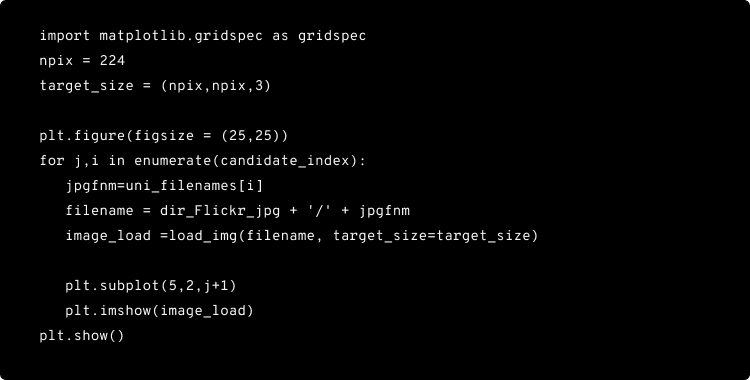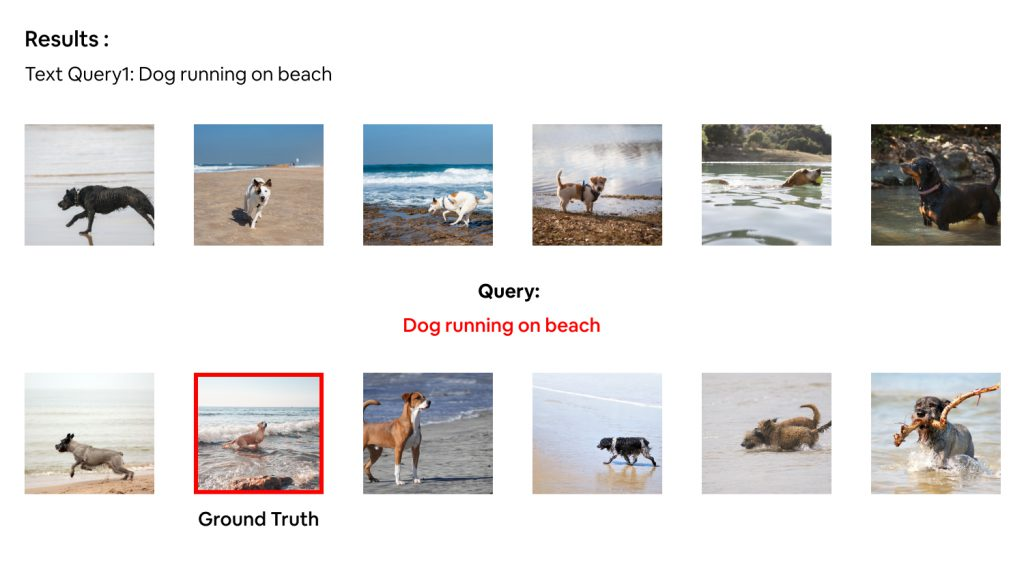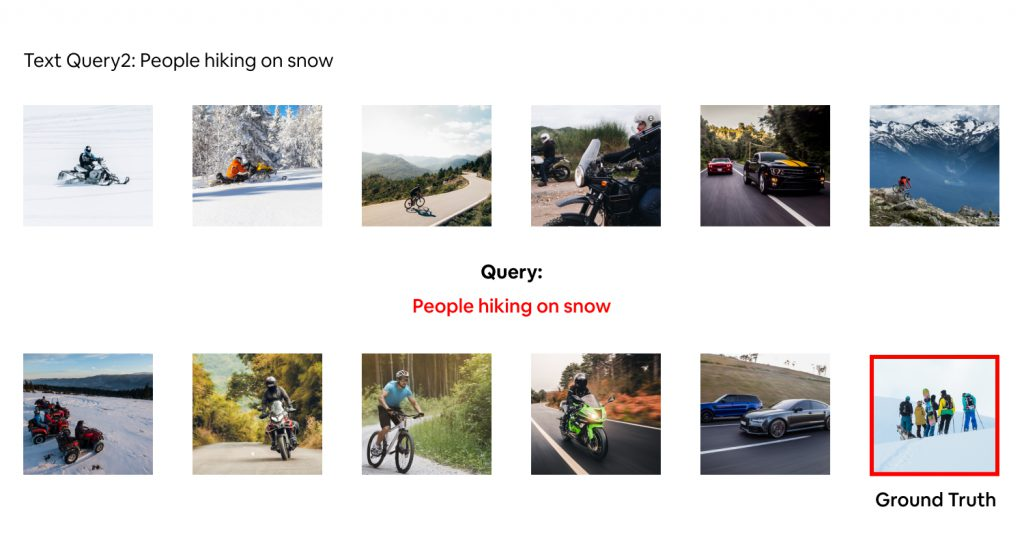## Conclusion

We have given a custom query to which we were able to retrieve the top 10 ranked images corresponding to the query entered. To analyze the performance, we have shown, both, success and failure scenarios. From the images above, we can consider Text Query 1 as a successful scenario and Text Query 2 as a failure scenario because, for Text Query 2, only one image that corresponds to the context has been retrieved. All other images are of different contexts. This tells that the proposed model doesn’t work for all cases and contexts. For better contextual mapping of image and text, attribute or instance-based mapping can be built, where we localize each object instance in an image to the matching context of a query.

Image retrieval techniques, text-based and content-based, are gaining popularity with the abundant growth of visual information in large digital databases in recent years. They also have wide applicability in areas such as medical, remote sensing, forensic, security, ecommerce, multimedia, etc. as they have become a very active research area for database management and Computer Vision.

Text-based image retrieval (TBIR) systems use language in the form of strings or concepts to search relevant images. Computer Vision and Deep Learning algorithms analyze the content in the query image and return results based on the best-matched content. With the rapid advancement in Computer Vision and Natural Language Processing(NLP), understanding the semantics of text and images becomes mandatory as Computer Vision trains computers to interpret and understand the visual world whereas NLP gives machines the ability to read, understand, and derive meaning from human languages. These machines accurately identify and classify objects by using digital images and analyze them with deep learning models.

With Computer Vision and Natural Language Processing(NLP) gaining a lot of momentum in the recent technological developments, cross-modal network learning for image-text similarity plays a very important role in the task of a query(image/text) based image retrieval as the application of image-text semantic mining can be harnessed using the cross-modal network. This blog focuses on text or referral, expression-based queries to rank and retrieve semantically similar images.

We attempted to explore the semantic similarity between the visual and text features that can be used to further research on object and phrase localization areas by building a cross-modal similarity network that uses Triplet loss to train and use Cosine similarity to generate K-similarity scores for a given text query. The dataset that we used for this problem is the Flickr8k dataset.

Let’s dive into building the machine learning pipeline that involves

– Data understanding and preparation
– Image and text embedding extraction
– Similarity network
– Triplet loss
– Training and evaluation

## Data understanding and preparation

We are using Flickr dataset as it is smaller and the image resolution considered is 224X224X3. Flickr dataset has two sections containing the images and their corresponding matching captions. The dataset contains a total of 8092 images in JPEG format with different shapes and sizes, of which 6000 are used for training, 1000 for the test, and 1000 for development. Flickr8k text contains text files describing the training set, test set. Flickr8k.token.txt contains 5 captions for each image i.e. total of 40460 captions.

Below are the code snippets for loading the data, checking the number of images and number of captions per image, plotting the image with its corresponding captions for 4 images.

– The total number of images is 8091.
– The number of captions per image is 5.

``````dir_Flickr_text = "flick_info/Flickr8k.token.txt"
dir_Flickr_jpg = "Flicker8k_Dataset"
jpgs = os.listdir(dir_Flickr_jpg)
print("The number of jpg flies in Flicker30k: {}".format(len(jpgs)))````````````## loading as dataframe
desc=dict()
text = text.iloc[1:,:]
df_new = text[text.iloc[:,2].notnull()]
print(df_new.iloc[:5,:])
return df_new``````
``````file = open(dir_Flickr_text,'r')
file.close()
datatxt = []
for line in text.split('\n'):
col = line.split('\t')
if len(col) == 1:
continue
w = col.split("#")
datatxt.append(w + [col.lower()])
df_txt = pd.DataFrame(datatxt,columns=["filename","index","caption"])
uni_filenames = np.unique(df_txt.filename.values)
print("The number of unique file names : {}".format(len(uni_filenames)))
print("The distribution of the number of captions for each image:")
Counter(Counter(df_txt.filename.values).values())
``````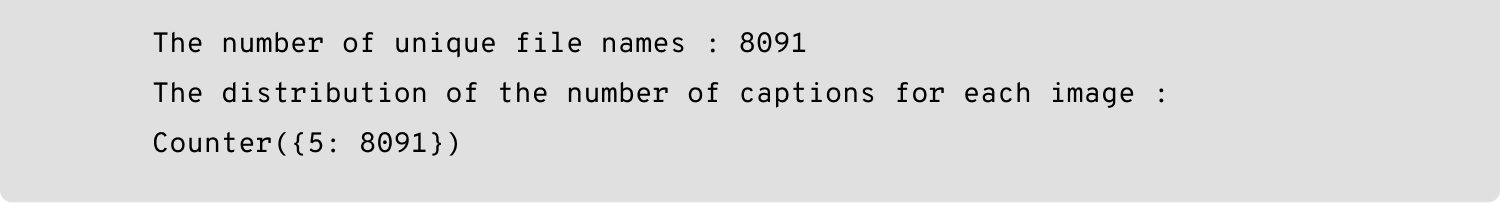``````npic = 5
npix = 224
target_size = (npix,npix,3)

count = 1
fig = plt.figure(figsize=(10,20))
for jpgfnm in uni_filenames[10:npic+10]:
filename = dir_Flickr_jpg + '/' + jpgfnm
captions = list(df_txt["caption"].loc[df_txt["filename"]==jpgfnm].values)

count += 1
plt.axis('off')
ax.plot()
ax.set_xlim(0,1)
ax.set_ylim(0,len(captions))
for i, caption in enumerate(captions):
ax.text(0,i,caption,fontsize=20)
count += 1
plt.show()
``````

The plot of 4 random images with corresponding captions are shown below:

##Get image embedding

Extraction of visual features is done by using a pre-trained model on ImageNet. ImageNet pretraining is an auxiliary task in Computer Vision problems. It is formally a project aimed at manually labeling and categorizing images into almost 22,000 separate object categories for the purpose of Computer Vision research. The state-of-the-art pre-trained networks included in the Keras core library demonstrate a strong ability to generalize images in real-life scenarios via transfer learning, such as feature extraction and fine-tuning.

There are various pre-trained models like VGG-19, RESNET, etc. Here we are using ResNet 50 for getting the image vectors. We need only the embedding vector, so we will not be using the entire network. Instead, we will use it until the “conv5_block3_3_conv” layer finally gives a 2048 sized embedding.

Below is the code snippet for extracting the image embeddings.

``````def get_image_codes(filenames, batch_size):
npix = 128
target_size = (npix,npix,3)
convnet = ResNet50(input_shape=(128,128,3),
include_top=False,
weights='imagenet')
convnet.summary()
def batch_gen(filenames, batch_size):
i = 0
while True:
f_names = filenames[i:batch_size+i]
#             print(f_names)
images = []
for jpgfnm in f_names:
filename = dir_Flickr_jpg + '/' + jpgfnm
images.append(img_data)
ib = np.asarray(list(images))
yield (ib)
i += batch_size

model = Model(inputs = convnet.input, outputs=convnet.get_layer("conv5_block3_3_conv").output)
batch_step = filenames.shape/batch_size
image_codes = model.predict(batch_gen(filenames, batch_size), steps=batch_step)
image_codes = image_codes.reshape(8091, 32768).astype(np.float32)

return image_codes

image_codes = get_image_codes(uni_filenames, 200)
print(image_codes.shape)
np.save('image_rep_resnet.npy', image_codes)
``````

In order to further optimize the performance of the model, instead of loading the entire image into the embedding model let us try and create mini-batches that yield a pool of image sets. Online preprocessing is applied on these mini-batches by converting the image into an n-dimensional array of pixel values, which are further normalized and passed into the CNN model that we considered from ResNet50 for prediction. The resultant output is the image embedding for the pool of images generated by the mini-batch creator. Online batch creation is the idea of yielding the preprocessed images on the fly based on the batch size mentioned.

## Get text embedding

For text embedding, individual words are represented as real-valued vectors in a predefined vector space where each word is mapped to one vector. The text embedding technique is often merged into the field of Deep Learning as the vector values are learned in a way that resembles a neural network. The distributed representation, based on the usage of words, captures their meaning by allowing words used in similar ways to result in having similar representations.

There are various pre-trained models, like Glove, SkipThoughts, etc, to get text embedding performed. The model we are considering is the Glove pre-trained model that derives the relationship between the words. It considers the global statistics-GloVe 300-dimensional vectors trained on the 42B token common crawl corpus.

The idea is to create a representation of words that capture the meanings, semantic relationships, and the contexts that they are used in. This will help us to achieve transfer learning. Transfer learning could be either about the words or about the embedding. Our main area of concentration is to obtain the embedding for the provided input captions.There are four functions used in this pipeline to extract the vectors of captions:

– loadGloveModel( ) – This function loads a pre-trained word embedding dictionary of 40000 words with 300 sized vectors each.
– Cap_tokenize(captions) – This function splits the sentences into tokens (words), removes punctuations, single letters, and finally joins them back as sentences.
– Text2seq (tokenized_captions) – This function converts captions into sequences of integers using Tensorflow functions like pad_sequences and text_to_sequences. The max vocabulary size is fixed as 4500 and the max length of any sequence is set as 30.
1. First, create a Tokenizer object with a vocabulary size of 4500.
2. The object is then trained on cleaned captions that return a word dictionary with an index based on the frequency of word occurrence in descending order.
3. Now, the captions are converted to integer sequences.
4. Use pad_sequences to have a max of 30 sequences in one row.
5. Create an embedding matrix of size (Vocab_size, 300 ), where 300 is the word vector dimension.

This function returns the word dictionary, tokenizer object, embedding matrix, and caption converted as sequences.

``````raw_captions = df_txt['caption']
tokenizer,wd,seq,embed_matrix = cap_embed(raw_captions)
embed_matrix.shape
```````````` print ("Loading Glove Model")
gloveFile = "glove.6B.300d.txt"
# open glove file and read its contents
with open(gloveFile, encoding="utf8" ) as f:

# initialise dictionalry model
model = {}
for line in content:
splitLine = line.split()
word = splitLine
embedding = np.array([float(val) for val in splitLine[1:]])
model[word] = embedding
return model
try:
g_model=model
except:
g_model = model
``````Below are the functions for text preprocessing and conversion of text to sequences:

``````def cap_tokenize(captions):
captions_raw  = []
table = str.maketrans('', '', string.punctuation)
for caps in captions:
tokens=caps.split()
tokens = [word.lower() for word in tokens]
tokens = [w.translate(table) for w in tokens]
tokens = [word for word in tokens if len(word)>1]
tokens = [word for word in tokens if word.isalpha()]
captions_raw.append(' '.join(tokens))
return captions_raw
def text2seq(captions):
t = Tokenizer(num_words=4500)
t.fit_on_texts(captions)
word_dict = t.word_index
vocab_size = len(word_dict) + 1

encoded_captions = t.texts_to_sequences(captions)
embedding_matrix = np.zeros((vocab_size,300))
count = 0
for word, i in word_dict.items():
embedding_vector = g_model.get(word)
if embedding_vector is not None:
embedding_matrix[i] = embedding_vector
else:
count +=1
# print(word)
def cap_embed(captions):
t_cap = cap_tokenize(captions)
#     v_cap = embedding_matrix(t_cap)
t,word_dict,seq,embed_matrix = text2seq(t_cap)
return t,word_dict,seq,embed_matrix``````

## Building Siamese network with triplet loss (similarity network)

A Siamese network, an artificial neural network, computes comparable output vectors by using the same weight while working sequentially in two different input vectors. Learning in such a kind of a twin network can be done by considering the triplet/contrastive loss function.

The similarity network with triplet loss accepts three branches of input – consisting of an anchor image, a negative caption, and a positive caption in the embedded form. This network is an improvised version of a contrastive loss model, where if given a single input, it learns about the correct pair of image and text as well as learns which of the image-text pairs are not suited.

Each branch will be passed through a series of transformations (applied by fully connected layers separated by rectified linear unit non-linearities) which will output the similarity scores based on the Euclidean distance metric, after the conversion to modality robust features. The training objective is to minimize the triplet loss function that applies a margin-based penalty to an incorrect caption when it gets ranked higher than the correct one describing the anchor image similarly for the given positive caption, i.e., the image gets ranked higher than the unrelated images.Below is the model for the image encoder:

##### Image Encoding

``````# Image Encoding
model_img = Sequential(name="Image_Encode")
model_img.summary()
``````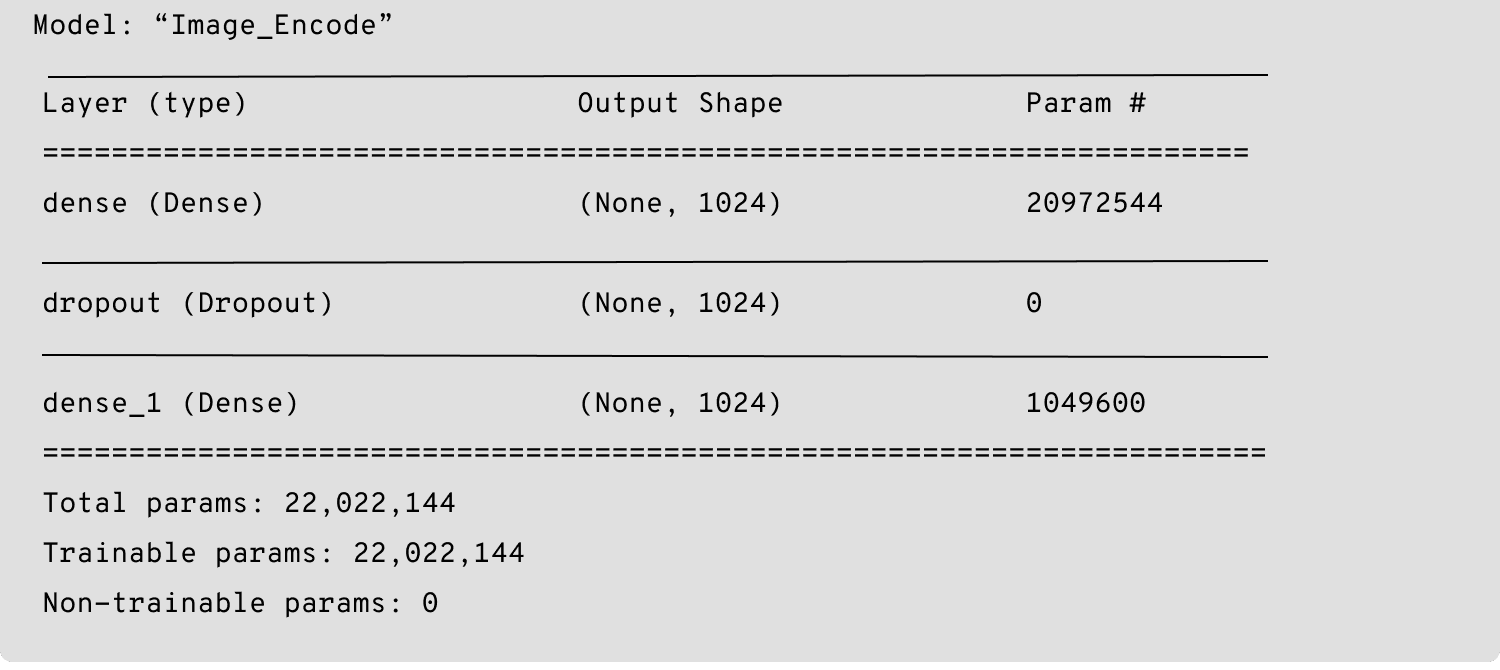Below is the model for text encoder:

##### Text Encode

``````# Text Encode
model_txt = Sequential(name='Text_encoder')
input_dim=len(wd)+1,
input_length=max_caps_len,
weights=[embed_matrix],
trainable=True))
model_txt.summary()
``````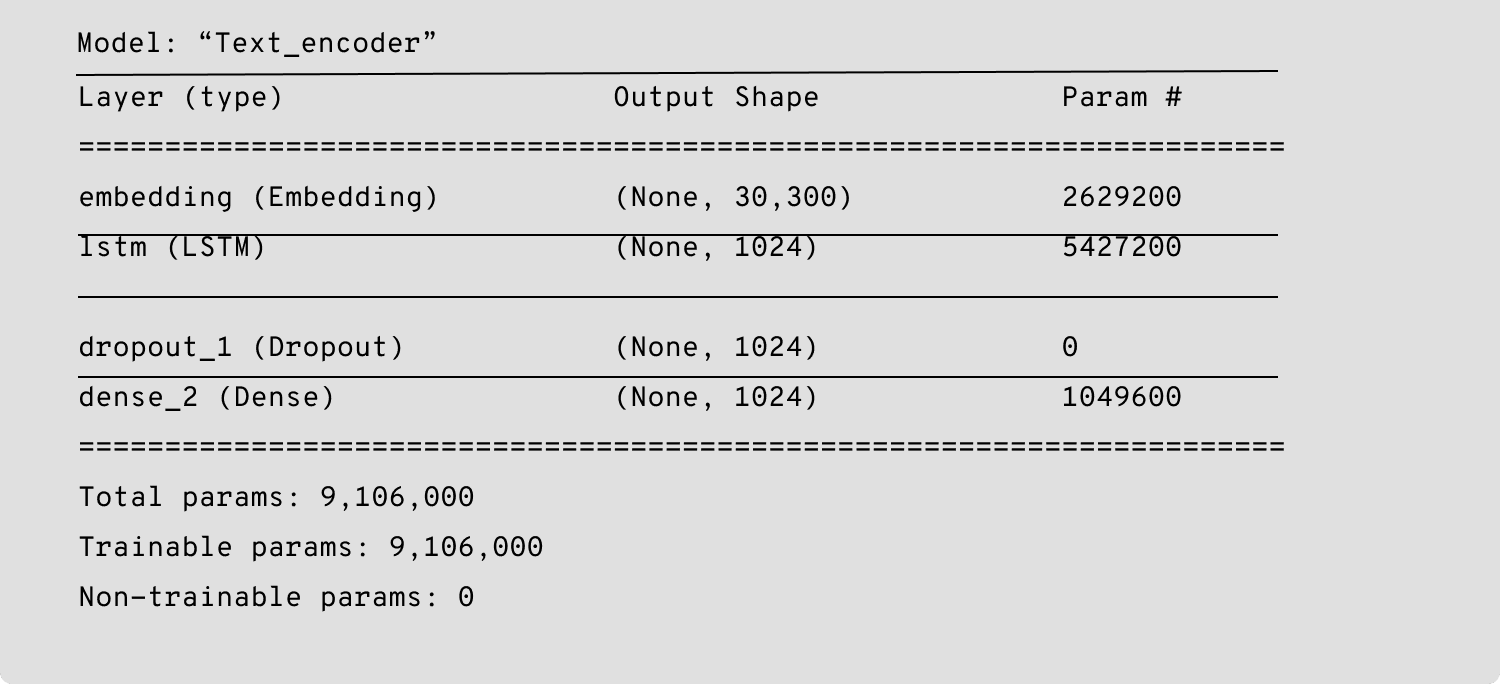Below is the Siamese model:

##### SiameseNet

``````# Siamese Net
anc_img = Input(shape=(img_in_dim,))
pos_txt = Input(shape=(max_caps_len,))
neg_txt = Input(shape=(max_caps_len,))

embed_anc = model_img(anc_img)
embed_pos = model_txt(pos_txt)
embed_neg = model_txt(neg_txt)

out = tf.keras.layers.concatenate([embed_anc, embed_pos, embed_neg], axis =1)
net = Model([anc_img,pos_txt,neg_txt], out)
net.summary()

``````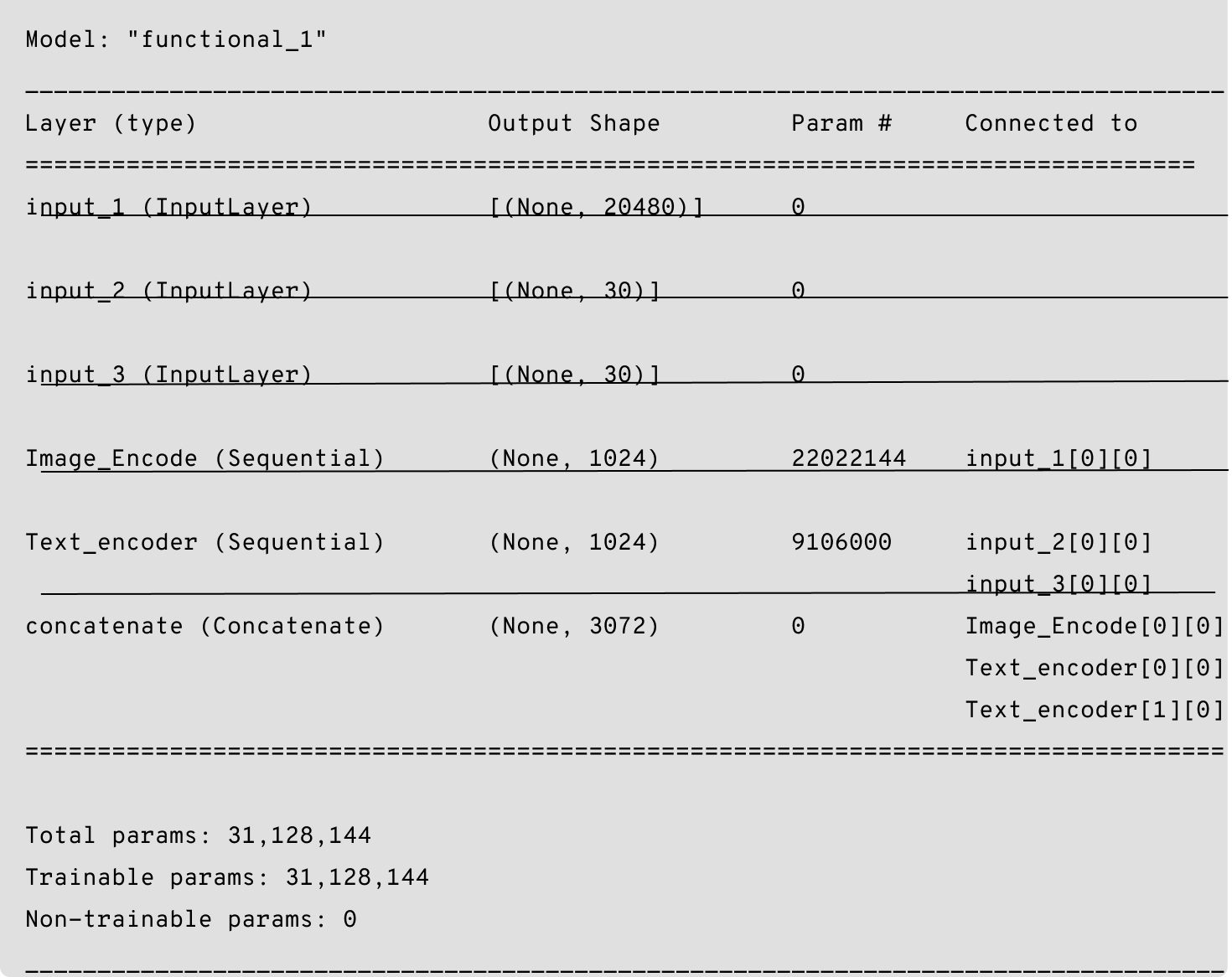## Triplet batching and Triplet loss

Given the anchor image “a”. Let p and n be the matching positive caption and the non-matching negative caption. We will calculate the euclidean distance between D1 (a, p) and D2 (a, n). We want the D1 value to be smaller than the distance value D2 and according to the definition of triplet loss, each negative caption “n” should be enforced with a margin of “m” which is called to be neighborhood updation. Based on the definition of triplet loss there are various options of selecting the triplets namely:
– Easy triplets
– Semi-hard triplets
– Hard triplets

An easy triplet has a loss of zero because D(a, p) + m will be less than D(a, n). Whereas, hard triplets are the ones in which the negative caption is closer to the anchor image than the positive caption because D(a, n) is less than D(a, P). Semi-hard triplets are the ones where the negative caption is not closer to the anchor image than the positive caption – which still has a positive loss associated with it because D(a, p) is less than D (a, n) which is less than D(a, p) + m.

The ultimate goal is – given a caption, a pool of images, and given a K value for recall, the model should be able to retrieve the most relevant images with lower loss.

Below is the code snippet for Triplet batching function (Easy triplets) and Triplet loss function-

``````def create_batch(batch_size,test=False):

anchors = np.zeros((batch_size,img_in_dim))
positives = np.zeros((batch_size,max_caps_len))
negatives = np.zeros((batch_size,max_caps_len))

if test:
files = test_files
else:
files = train_files
for i in range(batch_size):
img_id = files[np.random.randint(len(files))]
idx_a = np.where(uni_filenames==img_id)
idx_p = idx_a*5+np.random.randint(4)
a_img = image_codes[idx_a]
p_cap = seq[idx_p]
idx_n = idx_a*5-3
n_cap = seq[idx_n]
#         print(idx_a)
#         print(idx_p)
#         print(idx_n)
anchors[i]=a_img
positives[i]=p_cap
negatives[i]=n_cap

return [anchors,positives,negatives]
``````
``````## Triplet loss
alpha = 0.2
def triplet_loss(y_true,y_pred):
anc = y_pred[:, :out_dim]
pos = y_pred[:,out_dim:2*out_dim]
neg = y_pred[:,2*out_dim:]
dp = tf.sqrt(tf.reduce_sum(tf.square(anc-pos), axis=1))
dn = tf.sqrt(tf.reduce_sum(tf.square(anc-neg), axis=1))
L = tf.maximum(dp-dn+alpha, 0)

return tf.reduce_mean(L,0)``````
``````def data_gen(batch_size, emb_dim,test=False):
while True:
if test:
x = create_batch(batch_size,test=True)
else:
x = create_batch(batch_size)
#         print(x.shape)
#         print(x.shape)
#         print(x.shape)
y = np.zeros((batch_size, 3*emb_dim))
yield x,y``````

## Training the Similarity network

Now that we have built our siamese network and triplet dataset, it is time to train our model. The data consist of 8091 images with 5 captions per image and out of which, 6091 images and their captions are used for training.

The training parameters are:

``````batch_size = 64
img_in_dim = 20480
txt_in_dim = 300
out_dim = 1024
epoch =30
max_caps_len =30``````
``````steps_per_epochs = 6091/batch_size
train_gen = data_gen(batch_size,out_dim)
test_gen = data_gen(batch_size,out_dim,test=True)

plot_model(net,show_shapes=True, show_layer_names=True, to_file='sia_model.png')``````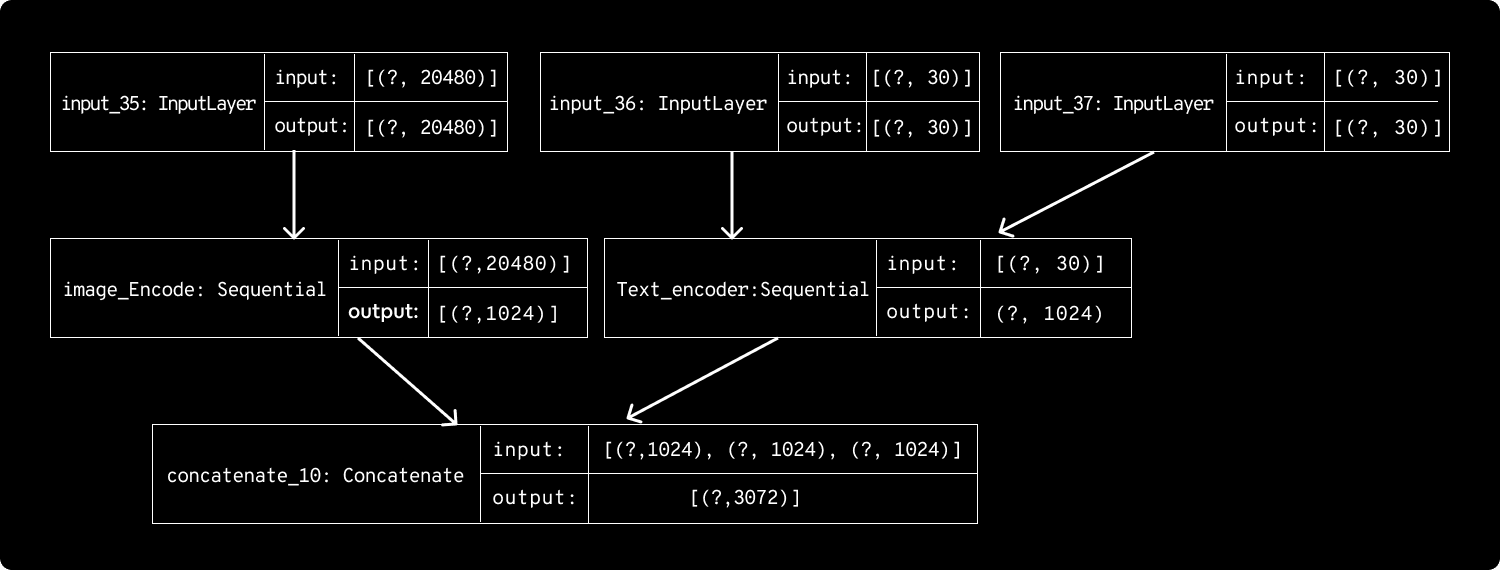``````history = net.fit_generator(
train_gen,
epochs=100,
steps_per_epoch=steps_per_epochs,
verbose=1,
)
``````

Following is the training loss plot :## Evaluation

As our model is now trained to understand the semantic similarity between an image and its corresponding captions, it is time to evaluate the model with test cases. For that, it is necessary to build a function user_input_text_embed( ) :

– The user can give any text query. The text is converted to a tokenized integer sequence. For this we use the same Tokenizer object we trained on train captions.
– The tokenized sequence is given to text_encode_model to predict its text embedding.

``````def text_emb(search_cap,tokenizer):
if type(search_cap) is list :
t_cap = cap_tokenize(search_cap)
else:
t_cap = cap_tokenize([search_cap])
encoded_captions = tokenizer.texts_to_sequences(t_cap)
return e_txt``````

These are some text inputs –

``````search_caption1 = "dog running on beach"
search_caption2 = "people hiking on snow"
search_caption3 = "a light-colored dog runs on the beach "
e_txt = text_emb(search_caption2,tokenizer)
``````

## Cosine Similarity Function:

We are now using cosine similarity to find the K-nearest image embeddings to the given text embedding.

``````# Find k nearest neighbour using cosine similarity
def find_k_nn(normalized_train_vectors,vec,k):
dist_arr = np.matmul(normalized_train_vectors, vec.T)
return np.argsort(-dist_arr.flatten())[:k]``````

Let us keep K =10, which means to output the top 10 images for a given text query.

``````k = 10
candidate_index = find_k_nn(img_emb, e_txt, k)
candidate_index
``````

Candidate index contains the index of 10 images :
Example: array([7778, 5830, 6500, 6534, 2893, 6290, 6354, 3239, 6948, 7762])

### Find the corresponding images using the indices:

``````for i in candidate_index:
print(uni_filenames[i])``````

Plotting Top K images in a grid:

``````import matplotlib.gridspec as gridspec
npix = 224
target_size = (npix,npix,3)

plt.figure(figsize = (25,25))
for j,i in enumerate(candidate_index):
jpgfnm=uni_filenames[i]
filename = dir_Flickr_jpg + '/' + jpgfnm

plt.subplot(5,2,j+1)
plt.show()
``````## Conclusion

We have given a custom query to which we were able to retrieve the top 10 ranked images corresponding to the query entered. To analyze the performance, we have shown, both, success and failure scenarios. From the images above, we can consider Text Query 1 as a successful scenario and Text Query 2 as a failure scenario because, for Text Query 2, only one image that corresponds to the context has been retrieved. All other images are of different contexts. This tells that the proposed model doesn’t work for all cases and contexts. For better contextual mapping of image and text, attribute or instance-based mapping can be built, where we localize each object instance in an image to the matching context of a query.

Image retrieval techniques, text-based and content-based, are gaining popularity with the abundant growth of visual information in large digital databases in recent years. They also have wide applicability in areas such as medical, remote sensing, forensic, security, ecommerce, multimedia, etc. as they have become a very active research area for database management and Computer Vision.